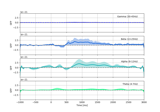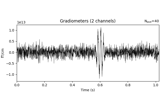# mne.baseline.rescale#

mne.baseline.rescale(data, times, baseline, mode='mean', copy=True, picks=None, verbose=None)[source]#

Rescale (baseline correct) data.

Parameters:
data`array`

It can be of any shape. The only constraint is that the last dimension should be time.

times

Time instants is seconds.

baseline`None` | `tuple` of length 2

The time interval to consider as “baseline” when applying baseline correction. If `None`, do not apply baseline correction. If a tuple `(a, b)`, the interval is between `a` and `b` (in seconds), including the endpoints. If `a` is `None`, the beginning of the data is used; and if `b` is `None`, it is set to the end of the interval. If `(None, None)`, the entire time interval is used.

Note

The baseline `(a, b)` includes both endpoints, i.e. all timepoints `t` such that `a <= t <= b`.

mode‘mean’ | ‘ratio’ | ‘logratio’ | ‘percent’ | ‘zscore’ | ‘zlogratio’

Perform baseline correction by

• subtracting the mean of baseline values (‘mean’)

• dividing by the mean of baseline values (‘ratio’)

• dividing by the mean of baseline values and taking the log (‘logratio’)

• subtracting the mean of baseline values followed by dividing by the mean of baseline values (‘percent’)

• subtracting the mean of baseline values and dividing by the standard deviation of baseline values (‘zscore’)

• dividing by the mean of baseline values, taking the log, and dividing by the standard deviation of log baseline values (‘zlogratio’)

copy`bool`

Whether to return a new instance or modify in place.

picks

Data to process along the axis=-2 (None, default, processes all).

verbose

Control verbosity of the logging output. If `None`, use the default verbosity level. See the logging documentation and `mne.verbose()` for details. Should only be passed as a keyword argument.

Returns:
data_scaled: `array`

Array of same shape as data after rescaling.

## Examples using `mne.baseline.rescale`#Explore event-related dynamics for specific frequency bands

Explore event-related dynamics for specific frequency bandsTime-frequency on simulated data (Multitaper vs. Morlet vs. Stockwell vs. Hilbert)

Time-frequency on simulated data (Multitaper vs. Morlet vs. Stockwell vs. Hilbert)